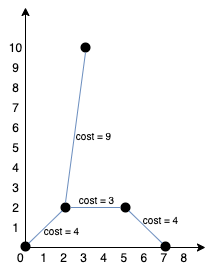# Min Cost to Connect All Points

published on 4/25/2022

You are given an array points representing integer coordinates of some points on a 2D-plane, where points[i] = [xi, yi].

The cost of connecting two points [xi, yi] and [xj, yj] is the manhattan distance between them: |xi - xj| + |yi - yj|, where |val| denotes the absolute value of val.

Return the minimum cost to make all points connected. All points are connected if there is exactly one simple path between any two points.

```Input: points = [[0,0],[2,2],[3,10],[5,2],[7,0]]
```

Output: 20## Explanation:

We can connect the points as shown above to get the minimum cost of 20. Notice that there is a unique path between every pair of points.

Constraints:

1 <= points.length <= 1000 -106 <= xi, yi <= 106 All pairs (xi, yi) are distinct.

```class Solution:    def minCostConnectPoints(self, points: List[List[int]]) -> int:        d, res = {(x, y): float('inf') if i else 0 for i, (x, y) in enumerate(points)}, 0        while d:            x, y = min(d, key=d.get)  # obtain the current minimum edge            res += d.pop((x, y))      # and remove the corresponding point            for x1, y1 in d:          # for the rest of the points, update the minimum manhattan distance                d[(x1, y1)] = min(d[(x1, y1)], abs(x-x1)+abs(y-y1))        return res
```

## Code walkthrough

Simple MST approch to solve approch to solve this problem.

Created with ❤️ by Avinash Sharma